The uniform stick of mass m length L is pivoted at the centre. In the equilibrium position shown in the figure, the identical light springs have their natural length. If the stick is turned through a small angle $\theta$, it executes SHM. The frequency of the motion is: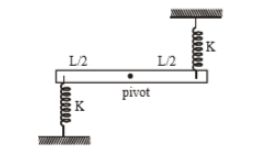(1) $\frac{1}{2\mathrm{\pi }}\sqrt{\frac{6K}{m}}$

(2) $\frac{1}{2\mathrm{\pi }}\sqrt{\frac{3K}{2m}}$

(3) $\frac{1}{2\mathrm{\pi }}\sqrt{\frac{3K}{m}}$

(4) None of these

Concept Questions :-

Angular SHM
High Yielding Test Series + Question Bank - NEET 2020

Difficulty Level:

If the displacement (x) and velocity v of a particle executing simple harmonic motion are related through the expression $4{v}^{2}=25-{x}^{2}$ then its time period is:

(1) $\mathrm{\pi }$

(2) 2$\mathrm{\pi }$

(3) 4$\mathrm{\pi }$

(4) 6$\mathrm{\pi }$

Concept Questions :-

Simple harmonic motion
High Yielding Test Series + Question Bank - NEET 2020

Difficulty Level:

Two simple pendulums have time periods T and $\frac{5T}{4}$. They start vibrating at the same instant from the mean position in the same phase. The phase difference between them when bigger pendulum completes one oscillation will be:

1. $\frac{\mathrm{\pi }}{6}$

2. $\frac{\mathrm{\pi }}{4}$

3. $\frac{\mathrm{\pi }}{3}$

4. $\frac{\mathrm{\pi }}{2}$

Concept Questions :-

Phasor diagram
High Yielding Test Series + Question Bank - NEET 2020

Difficulty Level:

A simple pendulum is oscillating without damping. When the displacement of the bob is less than maximum, its acceleration vector $\stackrel{\to }{a}$ is correctly shown in

 (1)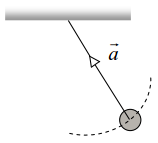(2)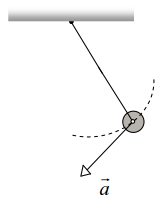(3)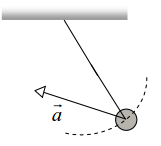(4)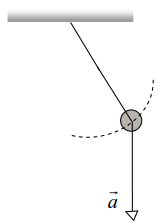Concept Questions :-

Simple harmonic motion
High Yielding Test Series + Question Bank - NEET 2020

Difficulty Level:

A particle undergoes SHM with a time period of 2 seconds. In how much time will it travel from its mean position to a displacement equal to half of its amplitude?

(1) $\frac{1}{2}s$

(2) $\frac{1}{6}s$

(3) $\frac{1}{4}s$

(4) $\frac{1}{3}s$

Concept Questions :-

Simple harmonic motion
High Yielding Test Series + Question Bank - NEET 2020

Difficulty Level:

There is a simple pendulum hanging from the ceiling of a lift. When the lift is stand still, the time period of the pendulum is T. If the resultant acceleration becomes g/4, then the new time period of the pendulum is

(1) 0.8 T

(2) 0.25 T

(3) 2 T

(4) 4 T

Concept Questions :-

Angular SHM
High Yielding Test Series + Question Bank - NEET 2020

Difficulty Level:

1.

2.

3.

4.

High Yielding Test Series + Question Bank - NEET 2020

Difficulty Level:

1.

2.

3.

4.  $\frac{2\mathrm{T}}{3}$

High Yielding Test Series + Question Bank - NEET 2020

Difficulty Level:

A block P of mass m is placed on a frictionless horizontal surface. Another block Q of same mass is kept on P and connected to the wall with the help of a spring of spring constant k as shown in the figure. μs is the coefficient of friction between P and Q. The blocks move together performing SHM of amplitude A. The maximum value of the friction force between P and Q is -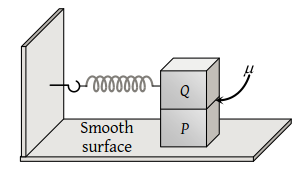(1) kA

(2) $\frac{kA}{2}$

(3) Zero

(4) μs mg

Concept Questions :-

Simple harmonic motion
High Yielding Test Series + Question Bank - NEET 2020

Difficulty Level:

which of the following figure represents damped harmonic motion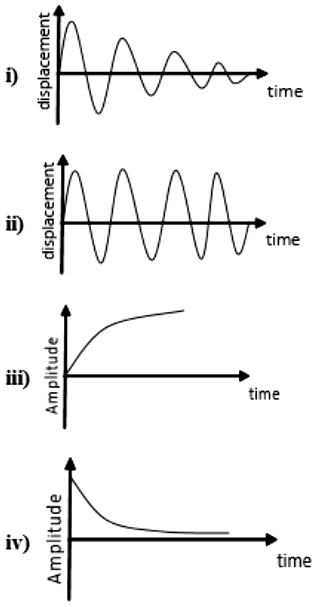1.  i and ii

2.  iii and iv

3.  i, ii, iii, and iv

4.  i, and  iv